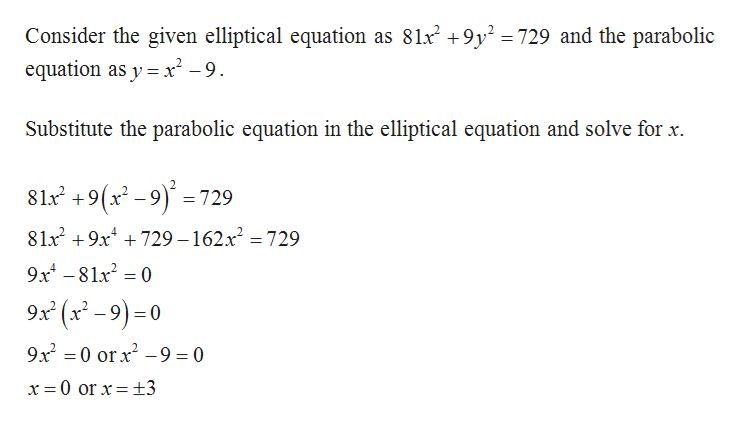# A planet follows an elliptical path described by 81x+9y= 729. A comet follows the parabolic path y x-might the comet intersect the orbiting planet?-9. WhereThe comet might intersect the planet's orbit at the points

Question
78 viewshelp_outlineImage TranscriptioncloseA planet follows an elliptical path described by 81x+9y= 729. A comet follows the parabolic path y x- might the comet intersect the orbiting planet? -9. Where The comet might intersect the planet's orbit at the points fullscreen
check_circle

Step 1help_outlineImage TranscriptioncloseConsider the given elliptical equation as 81x2 +9y2 =729 and the parabolic equation as y x-9 Substitute the parabolic equation in the elliptical equation and solve for x 81 +9(x-9)729 8lx2 9x729 -162x2 = 729 9x481x2 0 9x (x-9)0 9x2 0 orx29 = 0 11 x = 0 or x = +3 fullscreen

### Want to see the full answer?

See Solution

#### Want to see this answer and more?

Solutions are written by subject experts who are available 24/7. Questions are typically answered within 1 hour.*

See Solution
*Response times may vary by subject and question.
Tagged in

### Other